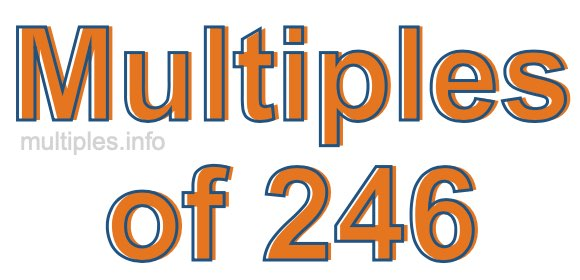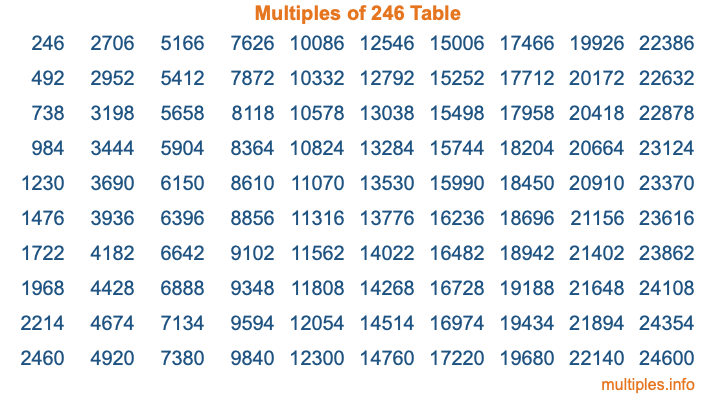Multiples of 246Welcome to the Multiples of 246 page. Here we will first teach you everything you will ever need to know about the multiples of 246, and then give you a study guide summary of everything we taught you to make sure you remember it all. Use this page to look up facts and learn information about the multiples of 246. This page will make you a multiples of two hundred forty-six expert!

Definition of Multiples of 246
Multiples of 246 are all the numbers that when divided by 246 equal an integer. Each of the multiples of 246 are called a multiple. A multiple of 246 is created by multiplying 246 by an integer.

Therefore, to create a list of multiples of 246, you start with 1 multiplied by 246, then 2 multiplied by 246, then 3 multiplied by 246, and so on for as long as you want. Thus, the list of the first five multiples of 246 is 246, 492, 738, 984, and 1230. To see a larger list of multiples of 246, see the printable image of Multiples of 246 further down on this page. We also have a category where you can choose any nth multiple of 246.

Multiples of 246 Checker
The Multiples of 246 Checker below checks to see if any number of your choice is a multiple of 246. In other words, it checks to see if there is any number (integer) that when multiplied by 246 will equal your number. To do that, we divide your number by 246. If the the quotient is an integer, then your number is a multiple of 246.

Is  a multiple of 246?

Least Common Multiple of 246 and ...
A Least Common Multiple (LCM) is the lowest multiple that two or more numbers have in common. This is also called the smallest common multiple or lowest common multiple and is useful to know when you are adding our subtracting fractions. Enter one or more numbers below (246 is already entered) to find the LCM.

Check out our LCM Calculator if you need more details about the Least Common Multiple or if you need the LCM for different numbers for adding and subtraction fractions.

nth Multiple of 246
As we stated above, 246 is the first multiple of 246, 492 is the second multiple of 246, 738 is the third multiple of 246, and so on. Enter a number below to find the nth multiple of 246.

th multiple of 246

Multiples of 246 vs Factors of 246
246 is a multiple of 246 and a factor of 246, but that is where the similarities end. All postive multiples of 246 are 246 or greater than 246. All positive factors of 246 are 246 or less than 246.

Below is the beginning list of multiples of 246 and the factors of 246 so you can compare:

Multiples of 246: 246, 492, 738, 984, 1230, etc.

Factors of 246: 1, 2, 3, 6, 41, 82, 123, 246

As you can see, the multiples of 246 are all the numbers that you can divide by 246 to get a whole number. The factors of 246, on the other hand, are all the whole numbers that you can multiply by another whole number to get 246.

It's also interesting to note that if a number (x) is a factor of 246, then 246 will also be a multiple of that number (x).

Multiples of 246 vs Divisors of 246
The divisors of 246 are all the integers that 246 can be divided by evenly. Below is a list of the divisors of 246.

Divisors of 246: 1, 2, 3, 6, 41, 82, 123, 246

The interesting thing to note here is that if you take any multiple of 246 and divide it by a divisor of 246, you will see that the quotient is an integer.

Multiples of 246 Table
Below is an image of the first 100 multiples of 246 in a table. The table is in chronological order, column by column. The first column has the first ten multiples of 246, the second column has the next ten multiples of 246, and so on.The Multiples of 246 Table is also referred to as the 246 Times Table or Times Table of 246. You are welcome to print out our table for your studies.

Negative Multiples of 246
Although not often discussed or needed in math, it is worth mentioning that you can make a list of negative multiples of 246 by multiplying 246 by -1, then by -2, then by -3, and so on, to get the following list of negative multiples of 246:

-246, -492, -738, -984, -1230, etc.

Multiples of 246 Summary
Below is a summary of important Multiples of 246 facts that we have discussed on this page. To retain the knowledge on this page, we recommend that you read through the summary and explain to yourself or a study partner why they hold true.

There are an infinite number of multiples of 246.

A multiple of 246 divided by 246 will equal a whole number.

246 divided by a factor of 246 equals a divisor of 246.

The nth multiple of 246 is n times 246.

The largest factor of 246 is equal to the first positive multiple of 246.

246 is a multiple of every factor of 246.

246 is a multiple of 246.

A multiple of 246 divided by a divisor of 246 equals an integer.

246 divided by a divisor of 246 equals a factor of 246.

Any integer times 246 will equal a multiple of 246.

Multiples of a Number
Here you can get the multiples of another number, all with the same attention to detail as we did for multiples of 246 on this page.

Multiples of
Multiples of 247
Did you find our page about multiples of two hundred forty-six educational? Do you want more knowledge? Check out the multiples of the next number on our list!